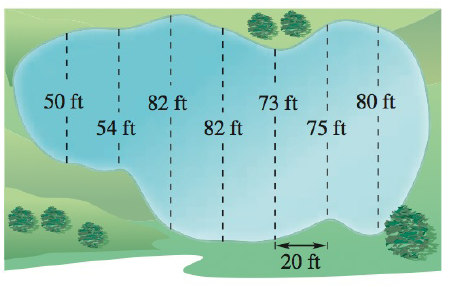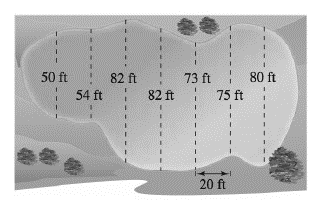Chapter 5.6, Problem 29E### Calculus: An Applied Approach (Min...

10th Edition
Ron Larson
ISBN: 9781305860919

#### Solutions

Chapter
Section### Calculus: An Applied Approach (Min...

10th Edition
Ron Larson
ISBN: 9781305860919
Textbook Problem
14 views

# Surface Area Use the Midpoint Rule to estimate the surface area of the pond shown in the figure.To determine

To calculate: The surface area of the pond shown in the figure below by application of the midpoint rule,Explanation

Given Information:

The figure showing the pond is provided below as,

Formula used:

The midpoint rule states that the midpoint (m) of each interval (a, b) is given by

m=a+b2.

Calculation:

The width of each area is 20 ft.

Calculate the midpoint of each of the intervals provided as,

[0,50],[50,54],[54,82],[82,82],[82,73],[73,75],[75,80],[80,]

Calculate the midpoint of the interval [0,50],

[0,50]=0+502=25

Calculate the midpoint of the interval [50,54],

[50,54]=50+542=52

Calculate the midpoint of the interval [54,82],

[54,82]=54+822=68

Calculate the midpoint of the interval [82,82]

### Still sussing out bartleby?

Check out a sample textbook solution.

See a sample solution

#### The Solution to Your Study Problems

Bartleby provides explanations to thousands of textbook problems written by our experts, many with advanced degrees!

Get Started